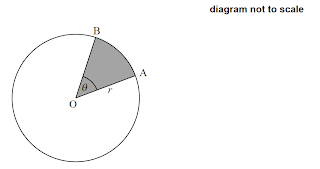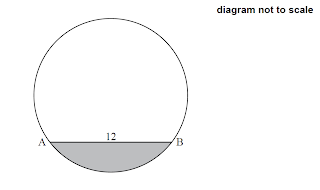# Arc length and Area of Sector (IB SL)

1 (IB/s1/2019/November/Paper2/q4)
[Maximum mark: 7]
The following diagram shows a right-angled triangle, $\mathrm{ABC}$, with $\mathrm{AC}=10 \mathrm{~cm}, \mathrm{AB}=6 \mathrm{~cm}$ and $\mathrm{BC}=8 \mathrm{~cm}$.
The points $\mathrm{D}$ and $\mathrm{F}$ lie on $[\mathrm{AC}]$. [BD] is perpendicular to [AC]. $B E F$ is the arc of a circle, centred at $A$. The region $R$ is bounded by [BD]. [DF] and are BEF.
 (a) Find BẢ. 
 (b) Find the area of $R$. 

2 (IB/sl/2019/May/paper2tz2/qu)
[Maximum mark: 7]
$\mathrm{OAB}$ is a sector of the circle with centre $\mathrm{O}$ and radius $r$, as shown in the following diagram.
The angle $\mathrm{AOB}$ is $\theta$ radians, where $0 < \theta < \frac{\pi}{2}$. The point $\mathrm{C}$ lies on $\mathrm{OA}$ and $\mathrm{OA}$ is perpendicular to $\mathrm{BC}$.
 (a) Show that $\mathrm{OC}=r \cos \theta$. 
 (b) Find the area of triangle $\mathrm{OBC}$ in terms of $r$ and $\theta$. 
 (c) Given that the area of triangle $\mathrm{OBC}$ is $\frac{3}{5}$ of the area of sector $\mathrm{OAB}$, find $\theta$. 

3 (IB/s1/2018/November/Paper1/q1)
[Maximum mark: 6]
The following diagram shows a circle with centre $\mathrm{A}$ and radius $6 \mathrm{~cm}$.
The points $\mathrm{B}, \mathrm{C}$, and $\mathrm{D}$ lie on the circle, and $\mathrm{B} \hat{\mathrm{A}} \mathrm{C}=2$ radians.
 (a) Find the area of the shaded sector. 
 (b) Find the perimeter of the non-shaded sector $\mathrm{ABDC}$. $$

 4 (IB/s1/2018/May/paper1tz2/q4) [Maximum mark: 7] The following diagram shows a circle with centre $\mathrm{O}$ and radius $r \mathrm{~cm}$.The points $\mathrm{A}$ and $\mathrm{B}$ lie on the circumference of the circle, and $\mathrm{AOB}=\theta$. The area of the shaded sector $\mathrm{AOB}$ is $12 \mathrm{~cm}^{2}$ and the length of are $\mathrm{AB}$ is $6 \mathrm{~cm}$. Find the value of $r$.

5 (IB/s1/2018/May/paper2tz1/q3)
[Maximum mark: 6]
The diagram shows a circle, centre $O$, with radius $4 \mathrm{~cm}$. Points $A$ and $B$ lie on the circumference of the circle and $\mathrm{AOOB}=\theta$, where $0 \leq \theta \leq \pi$.
 (a) Find the area of the shaded region, in terms of $\theta$.
 (b) The area of the shaded region is $12 \mathrm{~cm}^{2}$. Find the value of $\theta$. 

6 (IB/s1/2017/November/Paper1/q4)
[Maximum mark: 7 ]
The following diagram shows triangle $\mathrm{ABC}$, with $\mathrm{AB}=3 \mathrm{~cm}, \mathrm{BC}=8 \mathrm{~cm}$, and $\mathrm{ABC}=\frac{\pi}{3}$.
 (a) Show that $\mathrm{AC}=7 \mathrm{~cm}$. $$
 (b) The shape in the following diagram is formed by adding a semicircle with diameter $[\mathrm{AC}]$ to the triangle.

Find the exact perimeter of this shape. 

 7 (IB/s1/2017/May/paper2tz1/q5) [Maximum mark: 7] The following diagram shows the chord $[\mathrm{AB}]$ in a circle of radius $8 \mathrm{~cm}$, where $\mathrm{AB}=12 \mathrm{~cm}$.Find the area of the shaded segment.

8 (IB/s1/2017/May/paper2tz2/q1)
[Maximum mark: 6]
The following diagram shows a circle with centre $\mathrm{O}$ and radius $40 \mathrm{~cm}$.

The points $\mathrm{A}, \mathrm{B}$ and $\mathrm{C}$ are on the circumference of the circle and $\mathrm{AOC}=1.9$ radians.
 (a) Find the length of $\operatorname{arc} \mathrm{ABC}$.
 (b) Find the perimeter of sector $\mathrm{OABC}$.
 (c) Find the area of sector $\mathrm{OABC}$.

9 (IB/s1/2016/November/Paper2/q3)
[Maximum mark: 7]
The following diagram shows a circle, centre $\mathrm{O}$ and radius $r \mathrm{~mm}$. The circle is divided into five equal sectors.

One sector is $\mathrm{OAB}$, and $\mathrm{AO} \mathrm{B}=\theta$.
 (a) Write down the exact value of $\theta$ in radians. 

The area of sector $\mathrm{AOB}$ is $20 \pi \mathrm{mm}^{2}$.
 (b) Find the value of $r$. 
 (c) Find $\mathrm{AB}$. 

10 (IB/s1/2016/May/paper1tz2/q5)
[Maximum mark: 8]
The following diagram shows a triangle $\mathrm{ABC}$ and a sector $\mathrm{BDC}$ of a circle with centre $\mathrm{B}$ and radius $6 \mathrm{~cm}$. The points $\mathrm{A}, \mathrm{B}$ and $\mathrm{D}$ are on the same line.
$\mathrm{AB}=2 \sqrt{3} \mathrm{~cm}, \mathrm{BC}=6 \mathrm{~cm}$, area of triangle $\mathrm{ABC}=3 \sqrt{3} \mathrm{~cm}^{2}, \mathrm{ABC}$ is obtuse.
 (a) Find $\mathrm{ABC}$.
 (b) Find the exact area of the sector BDC. $$

11 (IB/s1/2015/November/Paper2/q1)
[Maximum mark: 6]
The following diagram shows a circle with centre $\mathrm{O}$ and radius $3 \mathrm{~cm}$.
Points $\mathrm{A}, \mathrm{B}$, and $\mathrm{C}$ lie on the circle, and $\mathrm{AOOC}=1,3$ radians.
 (a) Find the length of arc $\mathrm{ABC}$. 
 (b) Find the area of the shaded region. 

12 (IB/s1/2015/May/paper1tz1/q2)
[Maximum mark: 5 ]
The following diagram shows a circle with centre $\mathrm{O}$ and a radius of $10 \mathrm{~cm}$. Points $\mathrm{A}, \mathrm{B}$ and $\mathrm{C}$. lie on the circle.
Angle $\mathrm{AOB}$ is $1.2$ radians.
 (a) Find the length of are ACB.
 (b) Find the perimeter of the shaded region.

13 (IB/sl/2015/May/paper2tz2/q10)
[Maximum mark: 16]
The following diagram shows a square $\mathrm{ABCD}$, and a sector $\mathrm{OAB}$ of a circle centre $\mathrm{O}$, radius $r$. Part of the square is shaded and labelled $R$.
$A\hat OB=\theta,$ where $0.5\le\theta < \pi$.
 (a) Show that the area of the square $\mathrm{ABCD}$ is $2 r^{2}(1-\cos \theta)$.
 (b) When $\theta=\alpha$, the area of the square $\mathrm{ABCD}$ is equal to the area of the sector $\mathrm{OAB}$.

(i) Write down the area of the sector when $\theta=\alpha$.
(ii) Hence find $\alpha$.
 (c) When $\theta=\beta$, the area of $R$ is more than twice the area of the sector. Find all possible values of $\beta$.

(a) 0.927 (b) 8.05
(a) Show (b) $A=\frac{1}{2} r^{2} \sin \theta \cos \theta$ (c) $\theta=.830$
(a) Area $=36$ (b) Perimeter $=12 \pi$
 $r=4 \mathrm{~cm}$
(a) $A=8 \theta-8 \sin \theta$ (b) $\theta=2.27$
(a) Show (b) $11+\frac{7}{2} \pi$
 Area $=22.5$
(a) 76 (b) 156 (c) 1520
(a) $\theta=\frac{2 \pi}{5}$ (b) $r=10$ (c) $11.8$
(a) $\frac{5 \pi}{6}\left(150^{\circ}\right), \frac{\pi}{6}\left(30^{\circ}\right)$ (b) $3 \pi$
(a) $l=3.9$ (b) area $=9 \pi-5.85$
(a) $12$ (b) $\quad P=8+20 \pi$
(a) Show (b) (i) $\frac{1}{2} \alpha r^{2}$ (ii) $\alpha=0.511$ (c) $1.31<\beta<2.67$